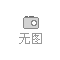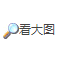400-6428-388

大量杨木原木求购！

距离结束时间： function TimeTo(dd){ var t = new Date(dd),//取得指定时间的总毫秒数 n = new Date().getTime(),//取得当前毫秒数 c = t - n;//得到时间差 if(c<=0){//如果差小于等于0 也就是过期或者正好过期，则推出程序 document.getElementById('timer').innerHTML ='本次采购已经结束'; clearInterval(window['ttt']);//清除计时器 return;//结束执行 } var ds = 60*60*24*1000,//一天共多少毫秒 d = parseInt(c/ds),//总毫秒除以一天的毫秒 得到相差的天数 h = parseInt((c-d*ds)/(3600*1000)),//然后取完天数之后的余下的毫秒数再除以每小时的毫秒数得到小时 m = parseInt((c - d*ds - h*3600*1000)/(60*1000)),//减去天数和小时数的毫秒数剩下的毫秒，再除以每分钟的毫秒数，得到分钟数 s = parseInt((c-d*ds-h*3600*1000-m*60*1000)/1000);//得到最后剩下的毫秒数除以1000 就是秒数，再剩下的毫秒自动忽略即可 document.getElementById('timer').innerHTML = ' <b>'+d+'</b> 天 <b>'+h+'</b></b> : <b>'+m+'</b> : <b class="ss">'+s+'</b>';//最后这句讲定义好的显示 更新到 ID为 timer的 div中 } (function(){ window['ttt']=setInterval(function(){ TimeTo('2021/10/02 23:59:59');//定义倒计时的结束时间，注意格 },1000);//定义计时器，每隔1000毫秒 也就是1秒 计算并更新 div的显示 })();

• 需求数量：
• 所在地： 江苏宿迁市
• 已有 97 人浏览••••• 暂无相关资料！

产品详细说明最新采购推荐

联系方式

注意:发布人未在本站注册，建议优先选择VIP会员